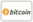Bookcover of Finite Dimensional Chebyshev Subspaces of Banach Spaces
Booktitle:

# Finite Dimensional Chebyshev Subspaces of Banach Spaces

## Extreme Points Metric Projection Chebyshev Subspaces (Uniquenes, Characterization & Existence)

Scholars' Press (2016-10-06 )eligible for voucher
ISBN-13:

### 978-3-659-84332-7

ISBN-10:
3659843326
EAN:
9783659843327
Book language:
English
Blurb/Shorttext:
A Chebyshev set is a subset of a normed linear space that admits unique best approximations. In 1853, the Russian mathematician Chebyshev asked the question: "can we represent any continuous function defined on [a,b] by a polynomial, of degree at most n, in such a way that the maximum error at any point in [a,b] is controlled?" Since then, the mathematicians have searched : why such a polynomial should exist? If it does, can we hope to construct it? If it exists, is it also unique? What happens if we change the measure of error? The aim of this book is to study finite dimensional Chebyshev subspaces of all classical Banach Spaces. In addition, you can find a valuable review for extreme points which are not found in books or articles. The main topics that are included in this book: Normed linear and Banach spaces, convexity, bounded linear operators, Hilbert spaces, topological vector spaces, Hahn-Banach theorems, reflexivity, w-topology and w*-topology, extreme points and sets, best approximation and proximinal sets, Chebyshev subspaces, metric projection, uniqueness and Characterization of best approximation, existence of Chebyshev subspaces and Chebyshev Subspaces of C[a, b].
Publishing house:
Scholars' Press
Website:
http://www.scholars-press.com
By (author) :
Mohammed Al Ghafri, Aref Kamal
Number of pages:
168
Published at:
2016-10-06
Stock:
Available
Category:
Other
Price:
79.90 €
Keywords:
convexity, functional analysis, Hilbert Spaces, Reflexivity, Chebyshev subspaces, Normed linear space, Banach space, Bounded linear operators, Topology and topological vector spaces, Hahn-Banach theorems, The w-topology and w*-topology, Extreme points, Best approximation, Metric projection, Uniqueness of best approximation, Existence of Chebyshev subspaces, The characterization of best approximation, Chebyshev subspaces of C(Q)### Categories